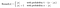# What is Stochastic Rounding?

It is just like any other rounding technique, but with the additional considerations of probability in order to make the decision of where to round.

# Why?

In the real world, it can come in handy in Machine Learning where low precision arithmetic is used repeatedly.

For example, I want to add 0.3 to 0 a hundred times. With normal rounding (rounding to nearest), I would get…

`100 * ( round(0.3) + 0 ) = 0`

With stochastic rounding, I would get 70% chance of rounding to 0, and 30% chance of rounding to 1. This means:

`100 * ( (30% * 1) + 0 )= 100 * 0.3= 30`

Which is what we would get if we didn’t do any rounding to the 0.3 number. Much more accurate than 0.

# How?

Here is the equation:From Wikipedia

Where:

• x = the number to be rounded
• ⌊x⌋ = is the floor of x (i.e. 1.2 =>1, 4.6 => 4)

# Examples

3.5 has a 50% chance to round to 3, and a 50% chance to round to 4

2.4 has a 60% chance to round to 2, and a 40% chance to round to 3

1.6 has a 40% chance to round to 1, and a 60% chance to round to 2

-2.1 has a 90% chance to round to -2, and a 10% chance to round to -3

-4.7 has a 30% chance to round to -4, and a 70% chance to round to -5

# Code Examples

Here is an example implementation in R from Heath Blackmon:

Here is an example implementation in C++ from myself (beware here its not exactly splitting 50% 50% on a 0.5 decimal value, but close enough for my application):

# Stochastic Rounding and You

Do you need it for your daily life? Nope.

I did need to learn the concept while reading this paper: Deep Learning with Limited Numerical Precision. Which is using this type of rounding to snap it to the nearest fixed-point resolution.

Welcome to a place where words matter. On Medium, smart voices and original ideas take center stage - with no ads in sight. Watch
Follow all the topics you care about, and we’ll deliver the best stories for you to your homepage and inbox. Explore
Get unlimited access to the best stories on Medium — and support writers while you’re at it. Just \$5/month. Upgrade